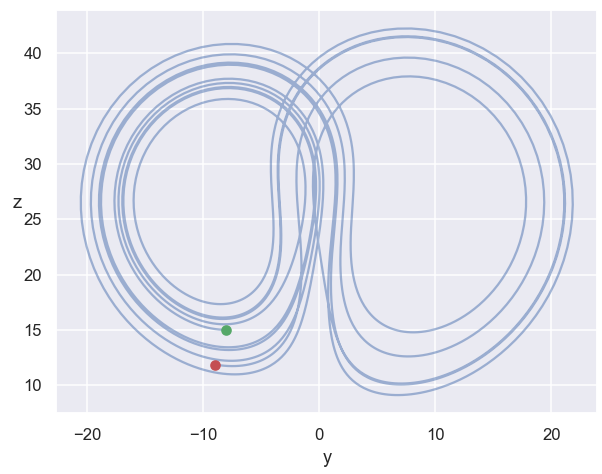# Homework 5¶

due 11:59pm Friday, March 19.

Complete illustrated "stories" to Gradescope. Code to UBlearns.

# 0. Find the mistake(s) in my implementation of RK4.¶

I noticed that my results were converging as $O(h)$ instead of as $O(h^4)$!

Find and fix the mistakes before using the code in 1. and 2.

# 1. Linear 2nd order shooting problem¶

Use the fixed RK4 code and just two exploratory shots to solve the BVP we looked at in class ("wild" DE) with $\alpha=1$ and $\beta=10$ as chosen by Chanon. Make the step size small enough that you think you have at least 8 digits of accuracy in your solution throughout the interval. Plot your two exploratory shots as well as the third shot that hits the target (all on the same axes). State the following for your approximate solutions: $y^\prime(0)$, $y(0.2)$, $y(1)$. (The last is supposed to be $\beta$, but state what you really got.)

# 2. Variational equations in Lorenz system¶

(a) Solve the variational equations for the Lorenz system IVP given in Homework #2 Q2 using the (fixed) RK4 code.Hint: Numpy functions reshape and append will be useful for packing and unpacking Y and V into/from a single 1D array of length $n+n^2$.

Calculate the matrix V(10.8), and its singular values (https://en.wikipedia.org/wiki/Singular_value_decomposition, np.linalg.svd).

(b) Interpret each of the singular values. One of them reflects the "butterfly effect" present in this system: sensitive dependence on initial conditions.

(Parenthetical note: An accurate computation of these singular values would involve re-orthogonalizing the matrix V(t) periodically to avoid collapse of its columns along the most rapidly expanding direction.)

In [ ]: